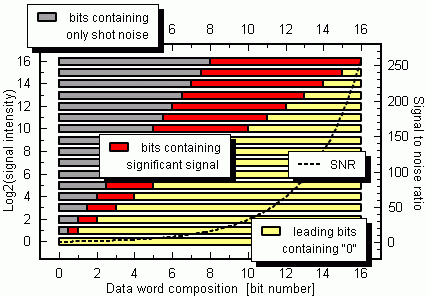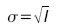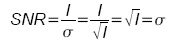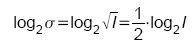# Shot noise limits the camera resolutionShot noise limits of the camera resolution

The photon noise, or more commonly called shot noise, is a basic physical characteristic of each light source that cannot be influenced, i.e. diminished, by any technical device. As described in section performance regimes light consists of single particles, photons. The lower the light level the smaller the number of photons which reach our detecor per unit of time. As a consequence there will not be a continuos illumination but a "hail like" bombardement by single photons and the image will appear granulous. The signal intensity, i.e. the number of arriving photons per unit of time, is stochastic and can be described by an average value and the appropriate fluctuations.

If I is the intensity of the light signal the Poisson distribution gives the signals fluctuations by the standard deviationThe average intensity value divided by the standard deviation of the fluctuations is called signal to noise ratio or SNRThe graphic below illustrates the consequence of this physical situation. Lets consider a 16 bit A/D conversion in the ICCD or EMCCD camera. This results in a 2-byte data word which is forwarded to the computer by camera. The left hand ordinate gives the actual signal intensity I, ranging from 20-1 to 216-1. The abscissa gives the numbers of the 16 bits of the data word, which is always divided into three basic categories:
- bits which contain the time resolved signal fluctuations, i.e. the shot noise,
- bits which contain the underlying non-fluctuant part of the signal, i.e. mainly the average intensity value and
- leading bits which only contain "0".

What we see is that the number of the bits which contain the signals fluctuations due to the shot noise always equals the number of bits which denominate the non-fluctuant part of the signal. This is simply due to equation (2) which may also be written asThis consideration tells that a 16 bit A/D conversion has no benefit in itself. If we would use e.g. a 12 bit A/D conversion, the quantization steps would be larger by the factor 2(16-12) = 16. The resolution would be decreased by this factor 16 which means that at the less-significant-bit end of the data word resolution would be lost. But the lsb end of the data word only contains the signals fluctuations, the shot noise. So, reducing the data word from 16 bit to 12 bit would result in a decreased resolution of the shot noise. The resolution of the significant non-fluctuant part of the signal would be preserved.

May be its hard to believe but in fact more bits in the A/D conversion can actually not increase the resolution of the data. There is only one chance to increase the resolution: go for higher intensity levels, i.e. longer time integration of the signal to reach higher signal to noise ratios. Of course there is a second chance, i.e. to use Stanford Computer Optics Dynamic Range Expansion system which strongly enhances real resolution after the A/D conversion and which is described in the following section.

To conclude: the usage of a high-bit A/D converter cannot increase the resolution of the aquired intensity data due to the physical shot noise restriction. High-bit A/D conversion is a valuable advertising point, not less, but also not more.